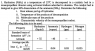# Temperature of the products of decomposition of hydrazine

Heresy42
Dear all,

## Homework Statement

For practice, I'm trying to solve an exercise I've found on the Internet. A screen shot of it is attached. I would like to be sure about my approach, especially when it comes to question 2.

Let's say, for the sake of simplification, that the reaction is as follows (no consideration of dissociation of ammonia):
5 N2H4 (g) -> 4 NH2 + 3 N2 + 6H2

2. The attempt at a solution

1/ Heat release per kg of hydrazine? I was thinking of computing the enthalpy of formation of the reaction to begin with: Delta Hf = 4 Delta Hf NH2 + 3 Delta Hf N2 + 6 Delta Hf H2 - 5 Delta Hf N2H4. The next step is to multiply by 5 mole and divide by 1 kg, right?

2/ Temperature of the products of decomposition, called T? What about the following, assuming that all hydrazine has been consumed: $$\Delta \ Hf (\mathrm{previously \ computed}) = 4 \int_{25}^{T} cp_{NH2} dT + 3 \int_{25}^{T} cp_{N2} dT + 6 \int_{25}^{T} cp_{H2} dT - 5 \int_{25}^{25} cp_{NH2} dT.$$
Is this equation right? Because I don't really how to tackle this question. What about the sign? Is it "Delta Hf ="or "- Delta Hf =".

3/ Molecular mass of the products MW? Is it possible to use the simple formula: MW = MWNH2*(4/13) + MWN2*(3/13) + MWH2*(6/13), with 13 = 4 + 3 + 2, assuming that all hydrazine has been consumed?

Thank you for checking my reasoning.

Regards.

#### Attachments

•Hydrazine decomposition.png
10.9 KB · Views: 1,127

JohnRC
Umm! -- The formula of ammonia is NH3. That makes a big difference, because the balancing of the equation was quite arbitrary the way you had it!

The appropriate equation is hydrazine --> ammonia + nitrogen (no hydrogen), and that can be uniquely balanced.

(2) The heat capacity approach will not work, because there is a difference between the chemical potential energy of the various species even at 0 K. Standard enthalpies of formation are tabulated for all of the species and relevant states at 25°C. These are what you should use, in conjunction with a balanced equation for the decomposition. That will get you the heat release for producing ammonia and nitrogen at 25°C

(3) You should then calculate the extra heat released or absorbed for transforming 30% of the ammonia to nitrogen and hydrogen. (It will actually be heat absorbed, so it will reduce the heat released). You then need to scale up from the number of mole in the balanced equation that you used to 1 kg hydrazine to get part (i) of the answer.

(4) You will then need to use the heat capacities to calculate the temperature rise of your mix of ammonia nitrogen and hydrogen. You can use either the quantities from your balanced equation or the 1 kg quantities; it does not matter as long as you are consistent. Part (ii) of the answer,

(5) An average molar mass (number average) should be calculated from the ammonia: nitrogen: hydrogen molar ratio of the product to get part (iii) of the answer.

(6) to get the answer to part (iv) you will need another equation relating the velocity to things like the temperature and the number average molar mass

Last edited:
Heresy42
Dear JohnRC,

You were right: in my previous post, I meant $$NH_3$$ instead of $$NH_2.$$

(1) So, the reaction should be rather:
$$3 N_2H_4 \rightarrow 4 NH_3 + N_2$$
$$1.2 NH_3 \rightarrow 0.6 N_2 + 1.8 H_2$$ (30% of $$4 NH_3$$ decomposes).

Since the final temperature is unknown, is using standard heat of formation at 25° the only way? Did you mean the following?
$$3 \Delta_r H_1 = 4 \times (- 46) + 1 \times 0 - 3 \times 55 = - 349$$ kJ/mol
$$3 \Delta_r H_2 = 0.6 \times 0 + 1.8 \times 0 - 1.2 \times (- 46) = 55.2$$ kJ/mol
$$3 \Delta H_3 = - 349 + 55.2 = - 293.8$$ kJ/mol
Hence: heat release per kg of hydrazine = 4 mol * 293.8 kJ/mol / 1kg = 1175.2 kJ/kg

(2) I'm not sure to have fully understood your next comment. What do you mean by balanced equation? What about the following?
$$3 N_2H_4 \rightarrow (4-1.2) NH_3 + N_2 + 1.2 NH_3 = 2.8 NH_3 + N_2 + 0.6 N_2 + 1.8 H_2 = 2.8 NH_3 + 1.6 N_2 + 1.8 H_2$$
$$- 293.8 = 2.8 \int_{25}^T cp_{NH_3} - 1.6 \int_{25}^T cp_{N_2} + 1.8 \int_{25}^T cp_{H_2} - 3 \int_{25}^{25} cp_{N_2H_4}$$
Then solving for the final temperature T of the mixture. But this doesn't sound natural to me, because -298.8 has been calculated for 25 degree C. So what is the right way to do it? Can you give me a mathematical expression?

(3) Your mentioned the ammonia: nitrogen: hydrogen molar ratio. Is the following right?
$$MW_{mixture} = \frac{2.8}{2.8+1.6+1.8} MW_{NH_3} + \frac{1.6}{2.8+1.6+1.8} MW_{N_2} + \frac{1.8}{2.8+1.6+1.8} MW_{H_2}$$

Thanks again.

Regards.